Thank you for visiting nature.com. You are using a browser version with limited support for CSS. To obtain the best experience, we recommend you use a more up to date browser (or turn off compatibility mode in Internet Explorer). In the meantime, to ensure continued support, we are displaying the site without styles and JavaScript.

# Optoelectronic Reservoir Computing

## Abstract

Reservoir computing is a recently introduced, highly efficient bio-inspired approach for processing time dependent data. The basic scheme of reservoir computing consists of a non linear recurrent dynamical system coupled to a single input layer and a single output layer. Within these constraints many implementations are possible. Here we report an optoelectronic implementation of reservoir computing based on a recently proposed architecture consisting of a single non linear node and a delay line. Our implementation is sufficiently fast for real time information processing. We illustrate its performance on tasks of practical importance such as nonlinear channel equalization and speech recognition and obtain results comparable to state of the art digital implementations.

## Introduction

The remarkable speed and multiplexing capability of optics makes it very attractive for information processing. These features have enabled the telecommunications revolution of the past decades. However, so far they have not been exploited insomuch as computation is concerned. The reason is that optical nonlinearities are very difficult to harness: it remains challenging to just demonstrate optical logic gates, let alone compete with digital electronics1. This suggests that a much more flexible approach is called for, which would exploit as much as possible the strengths of optics without trying to mimic digital electronics. Reservoir computing2,3,4,5,6,7,8,9,10, a recently introduced, bio-inspired approach to artificial intelligence, may provide such an opportunity.

Here we report the first experimental reservoir computer based on an opto-electronic architecture. As nonlinear element we exploit the sine nonlinearity of an integrated Mach-Zehnder intensity modulator (a well known, off-the-shelf component in the telecommunications industry) and to store the internal states of the reservoir computer we use a fiber optics spool. We report results comparable to state of the art digital implementations for two tasks of practical importance: nonlinear channel equalization and speech recognition.

Reservoir computing, which is at the heart of the present work, is a highly successful method for processing time dependent information. It provides state of the art performance for tasks such as time series prediction4 (and notably won a financial time series prediction competition11), nonlinear channel equalization4, or speech recognition12,13,14. For some of these tasks reservoir computing is in fact the most powerful approach known at present.

The central part of a reservoir computer is a nonlinear recurrent dynamical system that is driven by one or multiple input signals. The key insight behind reservoir computing is that the reservoir's response to the input signal, i.e., the way the internal variables depend on present and past inputs, is a form of computation. Experience shows that in many cases the computation carried out by reservoirs, even randomly chosen ones, can be extremely powerful. The reservoir should have a large number of internal (state) variables. The exact structure of the reservoir is not essential: for instance, in some works the reservoir closely mimics the interconnections and dynamics of biological neurons in a brain6, but many other architectures are possible.

To achieve useful computation on time dependent input signals, a good reservoir should be able to compute a large number of different functions of its inputs. That is, the reservoir should be sufficiently high-dimensional and its responses should not only depend on present inputs but also on inputs up to some finite time in the past. To achieve this, the reservoir should have some degree of nonlinearity in its dynamics and a “fading memory”, meaning that it will gradually forget previous inputs as new inputs come in.

Reservoir computing is a versatile and flexible concept. This follows from two key points: 1) many of the details of the nonlinear reservoir itself are unimportant except for the dynamic regime which can be tuned by some global parameters; and 2) the only part of the system that is trained is a linear output layer. Because of this flexibility, reservoir computing is amenable to a large number of experimental implementations. Thus proof of principle demonstrations have been realized in a bucket of water15 and using an analog VLSI chip16 and arrays of semiconductor amplifiers have been considered in simulation17. However, it is only very recently that an analog implementation with performance comparable to digital implementations has been reported: namely, the electronic implementation presented in18.

Our experiment is based on a similar architecture as that of18, namely a single non linear node and a delay line. The main differences are the type of non linearity and the desynchronisation of the input with respect to the period of the delay line. These differences highlight the flexibility of the concept. The performance of our experiment on two benchmark tasks, isolated digit recognition and non linear channel equalization, is comparable to state of the art digital implementations of reservoir computing. Compared to18, our experiment is almost 6 orders of magnitude faster and a further 2–3 orders of magnitude speed increase should be possible with only small changes to the system.

The flexibility of reservoir computing and its success on hard classification tasks makes it a promising route for realizing computation in physical systems other than digital electronics. In particular it may provide innovative solutions for ultra fast or ultra low power computation. In the Supplementary Material we describe reservoir computing in more detail and provide a road map for building high performance analog reservoir computers.

## Results

### A. Principles of Reservoir Computing

Before introducing our implementation, we recall a few key features of reservoir computing; for a more detailed treatment of the underlying theory, we refer the reader to Supplementary Material.

As is traditional in the literature, we will consider tasks that are defined in discrete time, e.g., using sampled signals. We denote by u(n) the input signal, whereis the discretized time; bythe internal states of the system used as reservoir; and bythe output of the reservoir. A typical evolution law foris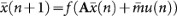, where f is a nonlinear function, A is the time independent connection matrix andis the time independent input mask. Note that in our work we will use a slightly different form for the evolution law, as explained below.

In order to perform the computation one needs a readout mechanism. To this end we define a subset xi(n), 0 ≤ iN − 1 (also in discrete time) of the internal states of the reservoir. It is these states which are observed and used to build the output. The time dependent output is obtained in an output layer by taking a linear combination of the internal states of the reservoir. The readout weights Wi are chosen to minimize the Mean Square Error (MSE) between the estimatorand a target function y(n):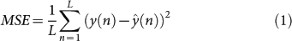over a set of examples (the training set). Because the MSE is a quadratic function of the Wi the optimal weights can be easily computed from the knowledge of xi(n) and y(n). In a typical run, the quality of the reservoir is then evaluated on a second set of examples (the test set). After training, the Wi are kept fixed.

### B. Principles of our implementation

In the present work we use an architecture related to that used in18 and to the minimum complexity networks studied in19. As in18, the reservoir is based on a non-linear system with delayed feedback (a class of systems widely studied in the nonlinear dynamics community, see e.g.20) and consists of a single nonlinear node and a delay loop. The information about the previous internal state of the reservoir up to some time T in the past is stored in the delay loop. After a period T of the loop, the entire internal state has been updated (processed) by the nonlinear node. In contrast to the work described in18, the nonlinear node in our implementation is essentially instantaneous. Hence, in the absence of input, the dynamics of our system can be approximated by the simple recursion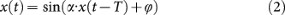where α (the feedback gain) and ϕ (the bias) are adjustable parameters and we have explicitly written the sine nonlinearity used in our implementation.

We will use this system to perform useful computation on input signals u(n) evolving in discrete time. As the system itself operates in continuous time, we need to define ways to convert input signal(s) to continuous time and to convert the system's state back to discrete time. The first is achieved by using a sample and hold procedure. We obtain a piecewise constant function u(t) of the continuous variable t : u(t) = u(n), nT′t < (n + 1)T′. The time T′T is taken to be less than or equal to the period T of the delay loop; when T′T we are in the unsynchronised regime (see below). To discretize the system's state, we note that the delay line acts as a memory, storing the delayed states of the nonlinearity. From this large-dimensional state space, we take N samples by dividing the input period T′ into N segments, each of duration θ and sampling the state of the delay line at a single point with periodicity θ. This provides us with N snapshots of the nonlinearity's response to each input sample u(n). From these snapshots, we construct N discrete-time sequences xi(n) = x(nT′ + (i + 1/2)θ) (i = 0, 1, …N − 1) to be used as reservoir states from which the required (discrete-time) output is to be constructed.

Without further measures, all such recorded reservoir states would be identical, so for computational purposes our system is one-dimensional. In order to use this system as a reservoir computer, we need to drive it in such a way that the xi(n) represent a rich variety of functions of the input history. It is often helpful9,19 to use an “input mask” that breaks the symmetry of the system. In18 good performance was improved by using a nonlinear node with an intrinsic time scale longer than the time scale of the input mask. In the present work we also use the “input mask”, but as our nonlinearity is instantaneous, we cannot exploit its intrinsic time scale. We instead chose to desynchronize the input and the reservoir, that is, we hold the input for a time T′ which differs slightly from the period T of the delay loop. This allows us to use each reservoir state at time n for the generation of a new different state at time n + 1 (unlike the solution used in18 where the intrinsic time scale of the nonlinear node makes the successive states highly correlated). We now explain these important notions in more detail.

The input mask m(t) = m(t + T′) is a periodic function of period T′. It is piecewise constant over intervals of length θ, i.e., m(t) = mj when nT′ + t < nT′ + (j + 1)θ, for j = 0, 1, …, N − 1. The values mj of the mask are randomly chosen from some probability distribution. The reservoir is driven by the product v(t) = βm(t)u(t) of the input and the mask, with β an adjustable parameter (the input gain). The dynamics of the driven system can thus be approximated by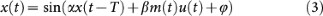It follows that the reservoir states can be approximated by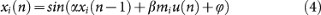when T′ = T (the synchronized regime); or more generally as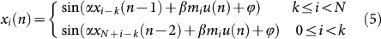when, (k {1, …, N − 1}) (the unsynchronized regime). In the synchronized regime, the reservoir states correspond to the responses of N uncoupled discrete-time dynamical systems which are similar, but slightly different through the randomly chosen mj. In the unsynchronized regime, with a desynchronization TT′ = , the state equations become coupled, yielding a much richer dynamics. With an instantaneous nonlinearity, desynchronisation is necessary to obtain a set of state transformations that is useful for reservoir computing. We believe that it will also be useful when the non linearity has an intrinsic time scale, as it provides a very simple way to enrich the dynamics.

In summary, by using an input mask, combined with desynchronization of the input and the feedback delay, we have turned a system with a one-dimensional information representation into an N-dimensional system.

### C. Hardware setup

The above architecture is implemented in the experiment depicted in Fig. 1. The sine nonlinearity is implemented by a voltage driven intensity modulator (Lithium Niobate Mach Zehnder interferometer), placed at the output of a continuous light source and the delay loop is a fiber spool. A photodiode converts the light intensity I(t) at the output of the fiber spool into a voltage; this is mixed with an input voltage generated by a function generator and proportional to m(t)u(t), amplified and then used to drive the intensity modulator. The feedback gain α is set by adjusting the average intensity I0 of the light inside the fiber loop with an optical attenuator. By changing α we can bring the system to the dynamical regime required. The nonlinear dynamics of this system have already been extensively studied, see21,22,23. The dynamical variable x(t) is obtained by rescaling the light intensity to lie in the interval [−1, +1] through x(t) = 2I(t)/I0 − 1. Then, neglecting the effect of the bandpass filter induced by the electronic amplifiers, the dynamics of the system is given by eq. (3) where α is proportional to I0. Equation (3), as well as the discretized versions thereof, eqs. (4) and (5), are derived in the supplementary material; the various stages of processing of the reservoir nodes and inputs are shown in Fig. 2.

In our experiment the round trip time is T = 8.504 µs and we typically use N = 50 internal nodes. The parameters α and β in eq. (3) are adjusted for optimal performance (their optimal value may depend on the task, see methods and supplementary material for details), while ϕ is set to 0, which seems to be the optimal value in all our experiments. The intensity I(t) is recorded by a digitizer and the estimatoris reconstructed offline on a computer.

We illustrate the operations of our reservoir computer in Fig. 3 where we consider a very simple signal recognition task. Here, the input to the system is taken to be a random concatenation of sine and square waves; the target function y(n) is 0 for a sine wave and 1 for a square wave. The top panel of Fig. 3 shows the input to the reservoir: the blue line is the representation of the input in continuous time u(t). In the bottom panel, the output of the network after training is shown with red crosses, against the desired output represented by a blue line. The performance on this task is essentially perfect: the Normalized Mean Square Error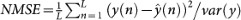reaches, which is significantly better than the results reported using simulations in17. (Note that, although reservoirs are usually trained using linear regression, i.e., minimizing the MSE, they are often evaluated using other error metrics. In order to be able to compare with previously reported results, we have adopted the most commonly used error metric for each task).

### D. Experimental results

We have checked the performance of this system extensively in simulations. First of all, if we neglect the effects of the bandpass filters and neglect all noise introduced in our experiment, we obtain a discretized system described by eq. (5) which is similar to (but nevertheless distinct from) the minimum complexity reservoirs introduced in19. We have checked that this discretized version of our system has performance similar to usual reservoirs on several tasks. This shows that the chosen architecture is capable of state of the art reservoir computing and sets for our experimental system a performance goal. Secondly we have also developed a simulation code that takes into account all the noises of the experimental components, as well as the effects of the bandpass filters. These simulations are in very good agreement with the experimentally measured dynamics of the system. They allow us to efficiently explore the experimental parameter space and to validate the experimental results. Further details on these two simulation models are given in the supplementary information.

We apply our optoelectronic reservoir to three tasks. These tasks are benchmarks which have been widely used in the reservoir computing community to evaluate the performance of reservoirs. They therefore allow comparison between our experiment and state of the art digital implementations of reservoir computing.

For the first task, we train our reservoir computer to behave like a Nonlinear Auto Regressive Moving Average equation of order 10, driven by white noise (NARMA10). More precisely, given the white noise u(n), the reservoir should produce an outputwhich should be as close as possible to the response y(n) of the NARMA10 model to the same white noise. The task is described in detail in the methods section. The performance is measured by the Normalized Mean Square Error (NMSE) between outputand target y(n). For a network of 50 nodes, both in simulations and experiment, we obtain a NMSE = 0.168 ± 0.015. This is similar to the value obtained using digital reservoirs of the same size. For instance a NMSE value of 0.15 ± 0.01 is reported in24 also for a reservoir of size 50.

For our second task we consider a problem of practical relevance: the equalization of a nonlinear channel. We consider a model of a wireless communication channel in which the input signal d(n) travels through multiple paths to a nonlinear and noisy receiver. The task is to reconstruct the input d(n) from the output u(n) of the receiver. The model we use was introduced in25 and studied in the context of reservoir computing in4. Our results, given in Fig. 4, are one order of magnitude better than those obtained in25 with a nonlinear adaptive filter and comparable to those obtained in4 with a digital reservoir. At 28 dB of signal to noise ratio, for example, we obtain an error rate of 1.3 · 10−4, while the best error rate obtained in25 is 4 · 10−3 and in4 error rates between 10−4 and 10−5 are reported.

Finally we apply our reservoir to isolated spoken digits recognition using a benchmark task introduced in the reservoir computing community in26. The performance on this task is measured using the Word Error Rate (WER) which gives the percentage of words that are wrongly classified. Performances reported in the literature are a WER of 0.55% using a hidden Markov model27; WERs of 4.3%26, of 0.2%12, of 1.3%19 for reservoir computers of different sizes and with different post processing of the output. The experimental reservoir presented in18 reported a WER of 0.2%. Our experiment yields a WER of 0.4%, using a reservoir of 200 nodes.

Further details on these tasks are given in the methods section and in the Supplementary Material.

## Discussion

We have reported the first demonstration of an opto-electronic reservoir computer. Our experiment has performance comparable to state of the art digital implementations on benchmark tasks of practical relevance such as speech recognition and channel equalization. Our work demonstrates the flexibility of reservoir computers that can be readily reprogrammed for different tasks. Indeed by re-optimizing the output layer (that is, choosing new readout weights Wk) and by readjusting the operating point of the reservoir (changing the feedback gain α, the input gain β and possibly the bias ϕ) one can use the same reservoir for many different tasks. Using this procedure, our experimental reservoir computer has been used successively for tasks such as signal classification, modeling a dynamical system (NARMA10 task), speech recognition and nonlinear channel equalization.

We have introduced a new feature in the architecture, as compared to the related experiment reported in18. Namely by desynchronizing the input with respect to the period of the reservoir we conserve the necessary coupling between the internal states, but make a more efficient use of the internal states as the correlations introduced by the low pass filter in18 are not necessary.

Our experiment is also the first implementation of reservoir computing fast enough for real time information processing. (We should point out that, after the submission of this manuscript, related results where reported in28). It can be converted into a high speed reservoir computer simply by increasing the bandwidth of all the components (an increase of at least 2 orders of magnitude is possible with off-the-shelf optoelectronic components). We note that in future realizations it will be necessary to have an analog implementation of the pre-processing of the input (digitisation and multiplication by the input mask) and of the post-processing of the output (multiplication by output weights), rather than the digital pre- and post-processing used in the present work.

From the point of view of applications, the present work thus constitutes an important step towards building ultra high speed optical reservoir computers. To help achieve this goal, in the supplementary material we present guidelines for building experimental reservoir computers. Whether optical implementations can eventually compete with electronic implementations is an open question. From the fundamental point of view, the present work helps understanding what are the minimal requirements for high level analog information processing.

## Methods

### Operating points

The optimal operating point of the experimental reservoir computer is task dependent. Specifically, if the threshold of instability (see Figure 1 in the supplementary material) is taken to correspond to 0 dB attenuation, then at the optimal operating point the attenuation varies between −0.5 and −4.2 dB. For the input gain, we set to 1 the minimum value of β that makes the Mach-Zehnder transmit the maximum light intensity when driven with an input equal to +1. Note that a small β value corresponds to a very linear regime, whereas a large β corresponds to a very non linear regime. At the optimal operating point, the multiplicative factor β for different tasks ranges from β = 0.55 to β = 10.5. For all tasks except the signal classification task the bias phase ϕ was set to zero. We did not try to optimize the bias phase ϕ. Details of the optimal operating points for each task are given in the supplementary material.

Auto Regressive models and Moving Average models and their generalization Nonlinear Auto Regressive Moving Average Models (NARMA), are widely used to simulate time series. The NARMA10 model is given by the recurrence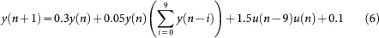where u(n) is a sequence of random inputs drawn from an uniform distribution over the interval [0, 0.5]. The aim is to predict the y(n) knowing the u(n). This task was introduced in29. It has been widely used as a benchmark in the reservoir computing community, see for instance19,24,30

### Nonlinear channel equalization

This task was introduced in25 and used in the reservoir computing community in4 and24. The input to the channel is an i.i.d. random sequence d(n) with values from {−3, −1, +1, +3}. The signal first goes through a linear channel, yielding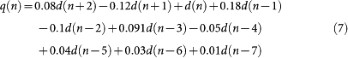It then goes through a noisy nonlinear channel, yielding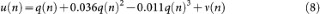where ν(n) is an i.i.d. Gaussian noise with zero mean adjusted in power to yield signal-to-noise ratios ranging from 12 to 32 db. The task is, given the output u(n) of the channel, to reconstruct the input d(n). The performance on this task is measured using the Symbol Error Rate, that is the fraction of inputs d(n) that are misclassified (Ref.24 used another error metric on this task).

### Isolated spoken digit recognition

The data for this task is taken from the NIST TI-46 corpus31. It consists of ten spoken digits (0…9), each one recorded ten times by five different female speakers. These 500 spoken words are sampled at 12.5 kHz. This spoken digit recording is preprocessed using the Lyon cochlear ear model32. The input to the reservoir uj(n) consists of an 86-dimensional state vector (j = 1,…, 86) with up to 130 time steps. The number of variables is taken to be N = 200. The input mask is taken to be a N × 86 dimensional matrix bij with elements taken from the the set {−0.1, +0.1} with equal probabilities. The product Σjbijuj(n) of the mask with the input is used to drive the reservoir. Ten linear classifiers(k = 0,…, 9) are trained, each one associated to one digit. The target function for yk(n) is +1 if the spoken digit is k and -1 otherwise. The classifiers are averaged in time and a winner-takes-all approach is applied to select the actual digit.

Using a standard cross-validation procedure, the 500 spoken words are divided in five subsets. We trained the reservoir on four of the subsets and then tested it on the fifth one. This is repeated five times, each time using a different subset as test and the average performance is computed. The performance is given in terms of the Word Error Rate, that is the fraction of digits that are misclassified. We obtain a WER of 0.4% (which correspond to 2 errors in 500 recognized digits).

## References

1. Caulfield, H. J. & Dolev, S. Why future supercomputing requires optics. Nature Photon. 4 261–263 (2010).

2. Jaeger, H. The “echo state” approach to analysing and training recurrent neural networks. Technical Report GMD Report 148, German National Research Center for Information Technology (2001).

3. Jaeger, H. Short term memory in echo state networks. Technical Report GMD Report 152, German National Research Center for Information Technology (2001).

4. Jaeger, H. & Haas, H. Harnessing nonlinearity: predicting chaotic systems and saving energy in wireless communication. Science 304, 78–80 (2004).

5. Legenstein, R. & Maass, W. New Directions in Statistical Signal Processing: From Systems to Brain, chapter What makes a dynamical system computationally powerful? pages 127–154. MIT Press (2005).

6. Maass, W., Natschlager, T. & Markram, H. Real-time computing without stable states: A new framework for neural computation based on perturbations. Neural Comput. 14, 2531–2560 (2002).

7. Steil, J. J. Backpropagation-decorrelation: online recurrent learning with O(N) complexity. 2004 IEEE International Joint Conference on Neural Networks 843–848 (2004)

8. Verstraeten, D., Schrauwen, B., D'Haene, M. & Stroobandt, D. An experimental unification of reservoir computing methods. Neural Netw. 20, 391–403 (2007).

9. Lukoševičius, M. & Jaeger, H. Reservoir computing approaches to recurrent neural network training. Computer Science Review 3, 127–149 (2009).

10. Hammer, B., Schrauwen, B. & Steil, J. J. Recent advances in efficient learning of recurrent networks. In: Proceedings of the European Symposium on Artificial Neural Networks pages 213–216 (2009).

11. Verstraeten, D., Schrauwen, B. & Stroobandt, D. Reservoir-based techniques for speech recognition. In: The 2006 IEEE International Joint Conference on Neural Network Proceedings 1050–1053 (2006).

12. Triefenbach, F., Jalalvand, A., Schrauwen, B. & Martens, J. Phoneme recognition with large hierarchical reservoirs. Advances in Neural Information Processing Systems 23, 1–9 (2010).

13. Jaeger, H., Lukosevicius, M., Popovici, D. & Siewert, U. Optimization and applications of echo state networks with leaky-integrator neurons. Neural Netw. 20, 335–52 (2007).

14. Fernando, C. & Sojakka, S. Pattern recognition in a bucket. In Banzhaf, W., Ziegler, J., Christaller, T., Dittrich, P. and Kim, J., editors, Advances in Artificial Life, volume 2801 of Lecture Notes in Computer Science 588–597. Springer Berlin / Heidelberg (2003).

15. Schürmann, F., Meier, K. & Schemmel, J. Edge of chaos computation in mixed-mode vlsi - a hard liquid. In: Advances in Neural Information Processing Systems. MIT Press (2005).

16. Vandoorne, K. et al. Toward optical signal processing using photonic reservoir computing. Opt. Express 16, 11182–92 (2008).

17. Appeltant, L. et al. Information processing using a single dynamical node as complex system. Nat. Commun. 2, 468 (2011).

18. Rodan, A. & Tino, P. Minimum complexity echo state network. IEEE T. Neural Netw. 22, 131–44 (2011).

19. Erneux, T. Applied Delay Differential Equations. (Springer Science + Business Media, 2009).

20. Larger, T., Lacourt, P. A., Poinsot, S. & Hanna, M. From flow to map in an experimental high-dimensional electro-optic nonlinear delay oscillator. Phys. Rev. Lett. 95, 1–4 (2005).

21. Chembo, Y. K., Colet, P., Larger, L. & Gastaud, N. Chaotic Breathers in Delayed Electro-Optical Systems. Phys. Rev. Lett. 95, 2–5 (2005).

22. Peil, M., Jacquot, M., Chembo, Y. K., Larger, L. & Erneux, T. Routes to chaos and multiple time scale dynamics in broadband bandpass nonlinear delay electro-optic oscillators. Phys. Rev. E 79, 1–15 (2009).

23. Rodan, A. & Tino, P. Simple deterministically constructed recurrent neural networks. Intelligent Data Engineering and Automated Learning - IDEAL 2010, 267–274 (2010).

24. Mathews, V. J. & Lee, J. Adaptive algorithms for bilinear filtering. Proceedings of SPIE 2296, 317–327 (1994).

25. Verstraeten, D., Schrauwen, B. & Stroobandt, D. Isolated word recognition using a liquid state machine. In: Proceedings of the 13th European Symposium on Artificial Neural Networks (ESANN) 435–440 (2005).

26. Walker, W. et al. Sphinx-4: a flexible open source framework for speech recognition. Technical report, Mountain View, CA, USA. (2004).

27. Larger, T. et al. Photonic information processing beyond Turing: an optoelectronic implementation of reservoir computing. Opt. Express 20, 3241–49 (2012).

28. Atiya, A. F. & Parlos, A. G. New results on recurrent network training: unifying the algorithms and accelerating convergence. IEEE T. Neural Netw. 11, 697–709 (2000).

29. Jaeger, H. Adaptive nonlinear system identification with echo state networks. In: Advances in Neural Information Processing Systems 8, 593–600. MIT Press (2002).

30. Texas Instruments-Developed 46-Word Speaker-Dependent Isolated Word Corpus (TI46), September 1991, NIST Speech Disc 7-1.1 (1 disc) (1991).

31. Lyon, R. A computational model of filtering, detection and compression in the cochlea. In: ICASSP ’82. IEEE International Conference on Acoustics, Speech and Signal Processing pages 1282–1285. IEEE (1982).

## Acknowledgements

We would like to thank J. Van Campenhout and I. Fischer for helpful discussions which initiated this research project. All authors would like to thank the researchers of the researchers of the Photonics@be network working on reservoir computing for numerous discussions over the duration of this project. The authors acknowledge financial support by Interuniversity Attraction Poles Program (Belgian Science Policy) project Photonics@be IAP6/10 and by the Fonds de la Recherche Scientifique FRS-FNRS.

## Author information

Authors

### Contributions

Y.P., J.D., B.S., M.H. and S.M. conceived the experiment. Y.P., F.D and A.S. performed the experiment and the numerical simulations, supervised by S.M. and M.H.. All authors contributed to the discussion of the results and to the writing of the manuscript.

## Ethics declarations

### Competing interests

The authors declare no competing financial interests.

## Rights and permissions

Reprints and Permissions

Paquot, Y., Duport, F., Smerieri, A. et al. Optoelectronic Reservoir Computing. Sci Rep 2, 287 (2012). https://doi.org/10.1038/srep00287

• Accepted:

• Published:

• ### Efficient reservoir computing using field programmable gate array and electro-optic modulation

• Prajnesh Kumar
• , Mingwei Jin
• , Ting Bu
• , Santosh Kumar
•  & Yu-Ping Huang

OSA Continuum (2021)

• ### Photonics for artificial intelligence and neuromorphic computing

• Bhavin J. Shastri
• , Alexander N. Tait
• , T. Ferreira de Lima
• , Wolfram H. P. Pernice
• , C. D. Wright
•  & Paul R. Prucnal

Nature Photonics (2021)

• ### Optoelectronic convolutional neural networks based on time-stretch method

• Yubin Zang
• , Minghua Chen
• , Sigang Yang
•  & Hongwei Chen

Science China Information Sciences (2021)

• ### Simulating self-learning in photorefractive optical reservoir computers

• Floris Laporte
• , Joni Dambre
•  & Peter Bienstman

Scientific Reports (2021)

• ### Predictive learning of multi-channel isochronal chaotic synchronization by utilizing parallel optical reservoir computers based on three laterally coupled semiconductor lasers with delay-time feedback

• Dongzhou Zhong
• , Hua Yang
• , Jiangtao Xi
• , Neng Zeng
• , Zhe Xu
•  & Fuqin Deng

Optics Express (2021)